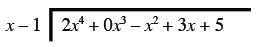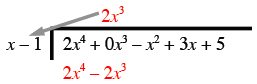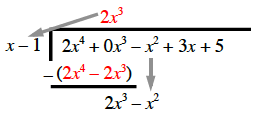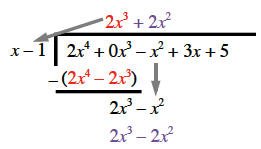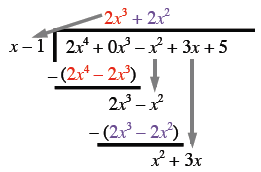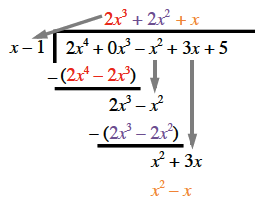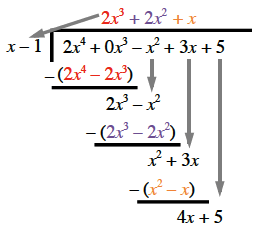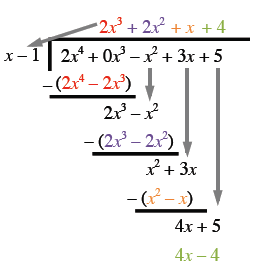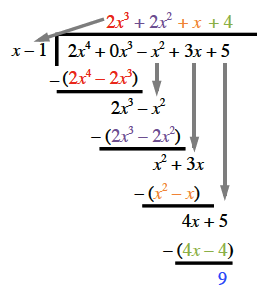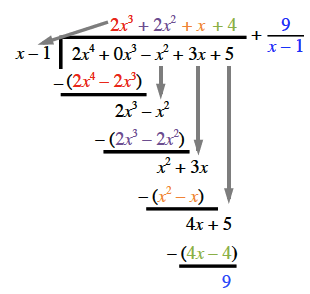### Home > PC > Chapter 7 > Lesson 7.2.4 > Problem7-82

7-82.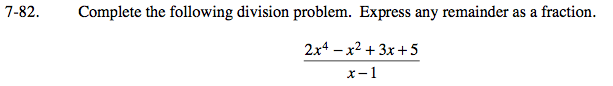Set up the problem.

Determine the first term of the quotient and multiply.

Subtract and bring the next term down.

Determine the next term of the quotient and multiply.

Subtract and bring the next term down.

Determine the next term of the quotient and multiply.

Subtract and bring the next term down.

Determine the next term of the quotient and multiply.

Subtract.

Write the remainder as a fraction.